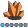ChuckK
Calcite | Level 5

## SGPLOT Aspect ratio

Hello All,

I wanted to see if there was anything new about changing the aspect ratio of the cell within SGPLOT. I know you can set the width and height in the template or by using the ODS GRAPHICS statement. As you know, though, that is for the entire output area. It does not give the same graph aspect ratio when there is a legend and when there's not, for example.

Is there a way to specifically set the width and height of the cell in SGPLOT.

Thanks for any help or suggestions.

Chuck

2 REPLIES 2

## SGPLOT Aspect ratio

Since nobody replied with an sgplot answer, here's a gplot alternative...

In gplot, you can specify the exact desired length of the axes (and thereby control the aspect ratio), using axis statements, such as:

axis1 length=2in;
axis2 length=4in;

proc gplot data=sashelp.class;
plot height*weight / vaxis=axis1 haxis=axis2;
run;

## Re: SGPLOT Aspect ratio

I do not know of how to specify an exact height and width, but there are the OFFSETMIN= and OFFSETMAX= options for the XAXIS and YAXIS statements, they allow you to controll how much space is used for the plotting, find a sample code below that illustrates this:

proc sgplot data=sashelp.class;
vbar age / group=sex;
xaxis offsetmax=0.3;

yaxis offsetmax=0.3;

keylegend / location=inside position=topright across=1;
run;
Discussion stats
• 2 replies
• 1344 views
• 0 likes
• 3 in conversation